Warning

# Support Vector Machines (SVM)

In the previous lab we have switched from the generative approach of classifier design to the discriminative one. Particularly, we have implemented the Perceptron algorithm. In this lab, we will continue examining the discriminative domain by implementing popular Support Vector Machines (SVM) classifier.

The perceptron algorithm has despite its simplicity several drawbacks. The first drawback is that it finds just some separating hyperplane (decision boundary), but ideally we would like to find the optimal separating hyperplane (at least in some sense). Another important drawback is, that perceptron cannot deal with noisy data.

The SVM are designed to handle both of these drawbacks. Given an annotated training data set $$\mathcal{T} = \{ (\mathbf{x}_i, y_i)\}_{i = 1}^{m} \quad,\ \text{where} \ \mathbf{x}_i \in \mathbb{R}^n,\ y_i \in \{-1, 1\}$$ the SVM primary task is formulated as $$\mathbf{w^*}, b^*, \xi_i^* = \arg\min_{\mathbf{w}, b, \xi_i} \left( \frac{1}{2} \| \mathbf{w} \|^2 + C \sum_{i=1}^{m}{\xi_i} \right)$$ subject to

\begin{align} \langle\mathbf{w}, \mathbf{x}_i\rangle + b & \ge +1 - \xi_i, \quad y_i = +1, \\ \langle\mathbf{w}, \mathbf{x}_i\rangle + b & \le -1 + \xi_i, \quad y_i = -1, \\ \xi_i & \ge 0,\ \forall i. \end{align}

Here, and in the following text, $\langle\mathbf{a}, \mathbf{b}\rangle$ denotes dot product $\mathbf{a}^\top \mathbf{b}$. As we can see, the SVM is posed purely as an optimization task.

The first term in the above optimisation task maximises so called margin, $2/||\mathbf{w}||$, by actually minimising $||\mathbf{w}||$. The margin is the distance between the separating hyperplane and the closest training points (intuitively, the further is the separating hyperplane from the training data, the better it generalises to unseen data). Thus the SVM are sometimes referred to as Maximum margin classifier. More detailed explanation can be found in [1, Section 3 - Linear Support Vector Machines].

The sum in the minimisation task is over so called slack variables, $\xi_i$. They allow some training points to be incorrectly classified (compare the separating hyperplane equation in the optimisation constraints with the one in Perceptron) by paying certain penalty controlled by the user specified constant $C$. This enables SVM to deal with noisy data (linearly non-separable due to noise).

Although intuitive, the primal task formulation is difficult to optimize. Thus, the parameters of the optimal separating hyperplane are usually sought by solving the dual problem.

The reason for solving rather the dual task is, that the separating hyperplane is fully specified only by a small number of points (compared to the usually very numerous training set), called support vectors. The derivation of the dual task can be found e.g. in  or . Note that to obtain the dual formulation, the student should be familiar with the Lagrange multipliers.

The dual task is formulated as $$\mathbf{\alpha^*} = \arg\max_{\mathbf{\alpha}} \left( \sum_{i=1}^{m} \alpha_i - \frac{1}{2} \sum_{i=1}^{m}\sum_{j=1}^{m}\alpha_i \alpha_j y_i y_j \langle\mathbf{x}_i, \mathbf{x}_j\rangle \right),$$

subject to

\begin{align} C \ge \alpha_i & \ge 0,\quad i = 1, \dots, m \\ \sum_{i=1}^{m} \alpha_i y_i & = 0 \end{align}

In case of hard-margin SVM, the transformation of the dual solution to the primal variables is done as follows:

\begin{align} \mathbf{w} & = \sum_{i=1}^{m} \alpha_i y_i \mathbf{x}_i, \\ b & = \frac{1}{| I |} \sum_{i \in I} (y_i - \sum_{j=1}^{m} \alpha_j y_j < \mathbf{x}_j, \mathbf{x}_i > ), \end{align}

where $I = \{i: 0 < \alpha_i \}$ denotes the set of support vector indices. This however is not correct for soft-margin SVM, where only the $\{i: 0 < \alpha_i < C\}$ indices are used to compute the bias (i.e. the support vectors directly on the margin). Unfortunately, it can easily happen that there is no vector directly on the margin. Because of this, the bias term has to be computed in a more complicated way (see libSVM paper (Section 4.1.5) or an “easy to follow” explanation (exercise 4 solution)). The bias computation code is provided in the assignment template (compute_bias).

Also for numerical reasons, it is more stable to compare $\alpha_i$ with a small non-zero constant (use $10^{-10}$) instead of zero.

Note that the original feature vectors $\mathbf{x}_i$ appear in the dual task in the form of dot product. This is very important and will be used in the next assignment!

Let us rewrite the dual task in matrix form:

$$\mathbf{\alpha^*} = \arg\max_{\mathbf{\alpha}} \left( \langle\mathbf{\alpha}, \mathbf{1}\rangle - \frac{1}{2} \mathbf{\alpha}^\top \mathbf{H} \mathbf{\alpha} \right),$$

subject to

\begin{align} \langle\mathbf{\alpha}, \mathbf{y}\rangle & = 0, \\ \mathbf{0} \le \mathbf{\alpha} & \le \mathbf{C} \end{align}

Having found the parameters of the optimal separating hyperplane, the classification of some data $\mathbf{x}$ using SVM is performed by a simple dot product and testing the sign of the result

\begin{align} h(\mathbf{x}; \mathbf{w}) & = \text{sign}(\langle\mathbf{x}, \mathbf{w}\rangle + b), \end{align}

which is very fast.

To fulfil this assignment, you need to submit these files (all packed in a single .zip file) into the upload system:

• answers.txt - answers to the Assignment Questions
• svm1.ipynb - a jupyter notebook for data initialisation, calling of the implemented functions and plotting of their results (for your convenience, will not be checked)
• svm1.py - containing the following implemented functions:
• my_svm - a function which implements the learning of a linear svm classifier
• classif_linear_svm - a function which classifies given data
• compute_test_error - a function computing mean error on test data from cross-validation
• compute_measurements_2d - a function which computes 2D features for given input data
• linear_svm.png, ocr_svm_tst.png, ocr_svm_classif.png - images specified in the tasks

Use template of the assignment. When preparing a zip file for the upload system, do not include any directories, the files have to be in the zip file root.

## Taks, Part 1: Soft margin SVM

1. Complete function my_svm( X, y, C, options ), which solves the SVM optimization task in its dual form. For solving the quadratic program use the gsmo function included in the template. Outputs of my_svm are parameters of the separating hyperplane $\mathbf{w},\ b$ and indices of support vectors sv_idx.

Hint: Look carefully at the gsmo help and compare it with the dual problem formulation.
Hint: Use the provided compute_bias function.

2. Complete the template function classif_linear_svm(X, model).
The function applies trained SVM on given data and outputs classification labels for all vectors in $X$.
$X$ is a matrix [$n \times m$], where $n$ is a dimensionality of features and $m$ is the number of examples and model is a structure with fields w and b. The output is vector (m, ) $\{-1, 1\}$ labels.

3. Try out function my_svm on toy dataset (data_33rpz_svm_toy.npz) and experiment with the values of parameter $C$.
Hint: You can limit maximal number of iterations for gsmo algorithm by passing tmax. Default value is inf (which might for linearly non-separable data cause infinite loop for certain values of $C$).

X = np.array([[1, 2, 1, -1, -1, -2], [1, 1, 2, -1, -2, -1]])
y = np.array([1, 1, 1, -1, -1, -1])
C = np.inf;

[w, b, sv_idx]  = my_svm(X, y, C)

print('\n', 'w: ', w, '\n', 'b: ', b, '\n', 'sv_idx:', sv_idx)
# -> t=1, KKTviol=2.000000, tau=0.250000, tau_lb=0.000000, tau_ub=inf, Q_P=-0.250000
# -> w:  [0.5 0.5]
# -> b:  0.0
# -> sv_idx: [0 3]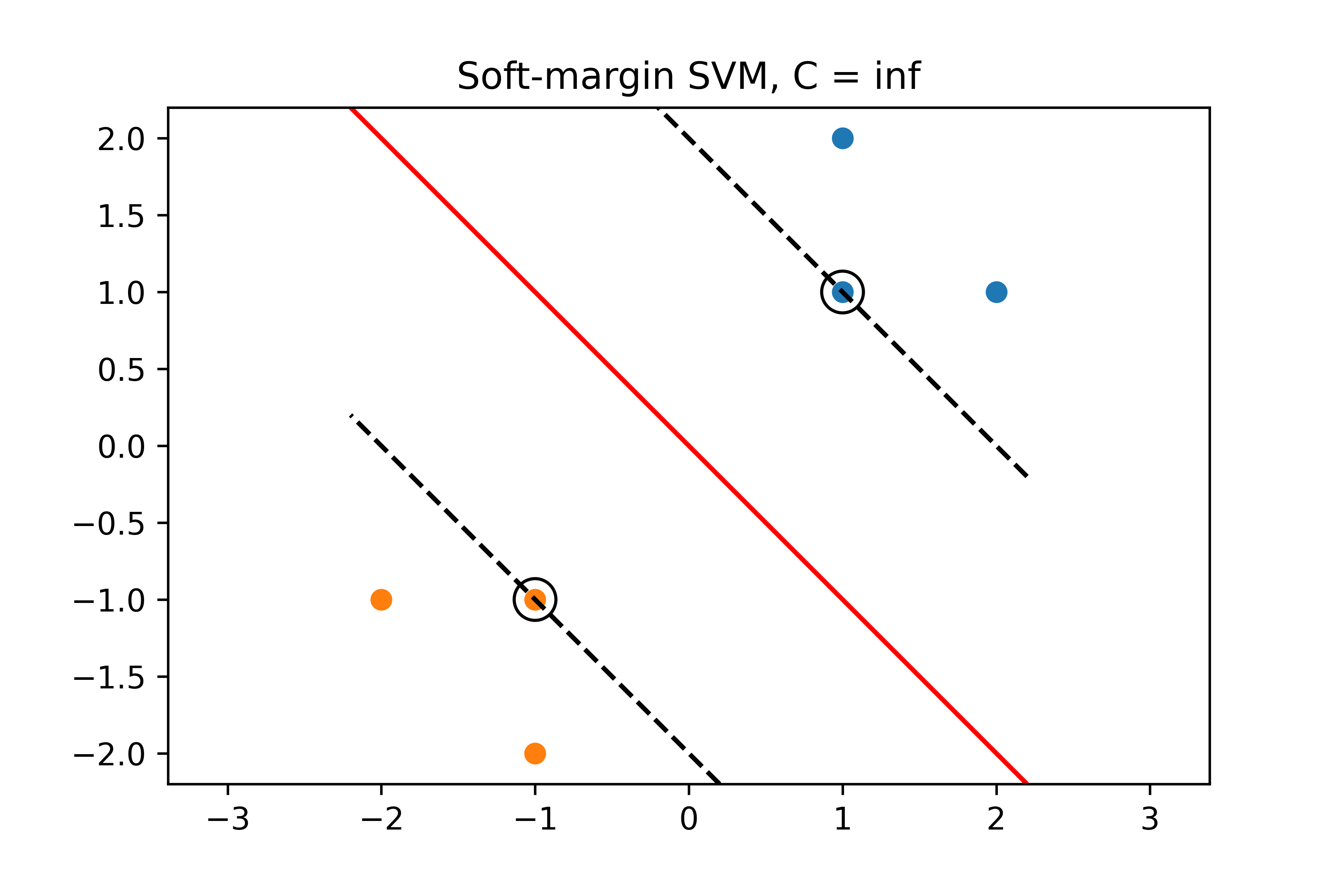4. Set the value of $C$ such that the resulting classifier has zero error on the training dataset (i.e. the data which you have used for training the SVM - data_33rpz_svm_toy.npz), keep the indices of support vectors in this case. You will need them to answer one of the assignment questions.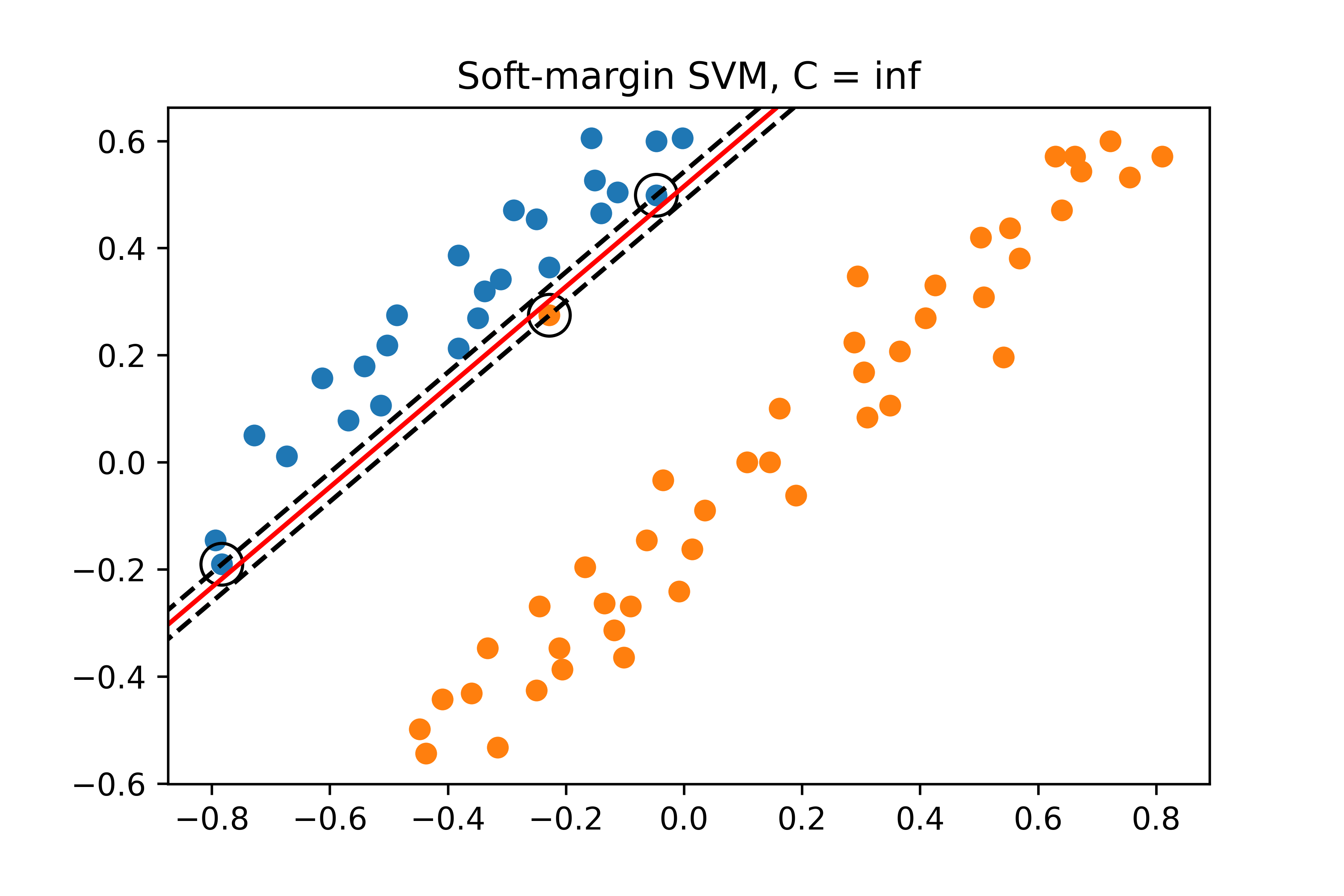Hint 1: You can use function classif_lin_svm and compare its output with the vector $y$ used for training the SVM.
Hint 2: For plotting you can use the functions plot_points and plot_boundary provided in the template.
5. Plot results for $C = 1$ and emphasize the support vectors. Save the figure linear_svm.png and submit it with your solution.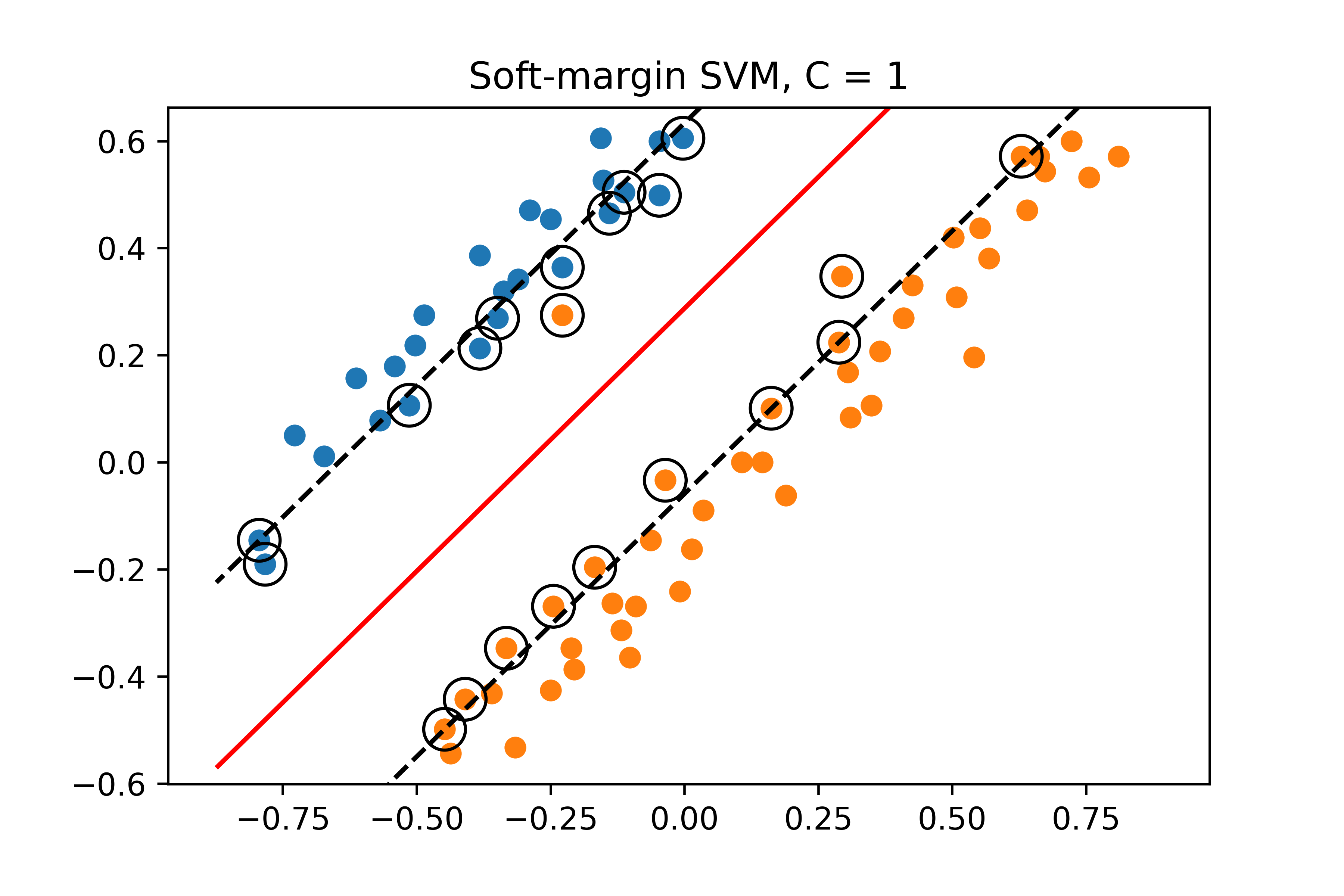## Tasks, Part 2: Optimal parameter selection

Similarly to the Parzen Window task, the optimal value of the hyper-parameter $C$ can be found using the cross-validation technique. To measure the quality of the parameter, we will compute the average error on test data overall folds and select such a value of $C$, which minimizes this error.

Do the following:

1. Prepare the OCR data for SVM training:
1. Complete the template function compute_measurements_2d(data).
The function computes 2D features from the images, and you need to fill in the necessary normalization (it is needed for gsmo to converge). The output of this function is
• $X$ is a matrix $2 \times m$, $X = [\mathbf{a}; \mathbf{b}]$, $\mathbf{a} = [a_1, \dots, a_m]$, $\mathbf{b} = [b_1, \dots, b_m]$
• $a_i =$ (sum of pixel intensities in the left half of the image i) - (sum of pixel intensities in the right half of the image i)
• $b_i =$ (sum of pixel intensities in the top half of the image i) - (sum of pixel intensities in the bottom half of the image i)
• Normalize both $\mathbf{a},\ \mathbf{b}$ as follows:
$\mathbf{c}_{\text{norm}} = \frac{\mathbf{c} - \mathbf{\bar{c}}}{\sigma_{\mathbf{c}}}, \quad c \in \{\mathbf{a}, \mathbf{b} \},$
where $\mathbf{\bar{c}}$ denotes the average of $\mathbf{c}$ and $\sigma_{\mathbf{c}}$ denotes the standard deviation of $\mathbf{c}$. Normalization of data is very important. The normalization which we use here is referred to as standardization and is commonly used in machine learning. Note that by this normalization, the feature points are transformed in such way, that they have zero mean and unit variance. When classifying new data you should first standardize them with the same $\mathbf{\bar{c}}$ and $\sigma_{\mathbf{c}}$.
• $y$ is a (m, ) np.array with values $\{-1, 1\}$
Hint: Labels that originally had the value 2 will now have value -1.
Hint: Carefully work with datatypes. Input images are stored as uint8 (numpy array). It may cause unwanted behaviour within the sum.

2. Estimate the optimal value of the parameter $C$ using the 10-fold cross-validation. The procedure is as follows:
1. Split the training set into 10 subsets using the crossval function (provided in template).

2. Complete the template function compute_test_error(itrn, itst, X, y, C).
The function trains an SVM classifier on $X(\text{itrn{i}})$ and computes the error $\text{Err(i)}$ (i.e. fraction of miss-classifications) on $X(\text{itst{i}})$. The output is the mean test error $\text{TstErr}$ over all 10 folds.

3. Use the function compute_test_error to perform cross-validation for $C \in \{0.001, 0.01, 0.1, 1, 10 \}$

4. Find the optimal value of $C$, i.e. such $C$ from the given set that minimizes the average test error. You will need this value in the questions below.

3. Compute the classification error of the trained SVM classifier on the test data. You will need this value in the questions below.

4. Plot the optimal separating hyperplane (optimal $C$) together with the test data in one plot and save it as ocr_svm_tst.png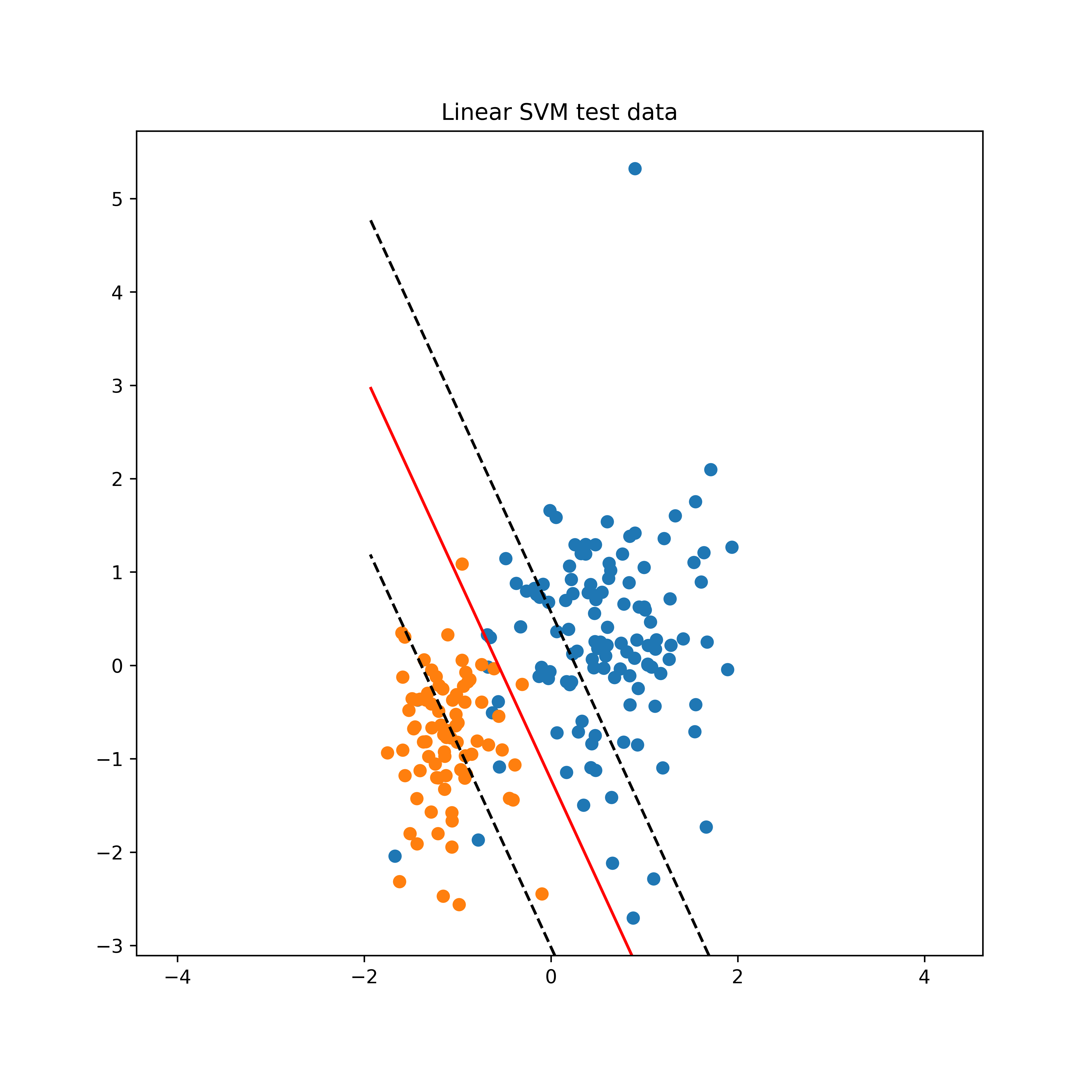5. Show the classification of the letters with the optimal classifier as in the previous labs and save it as ocr_svm_classif.png.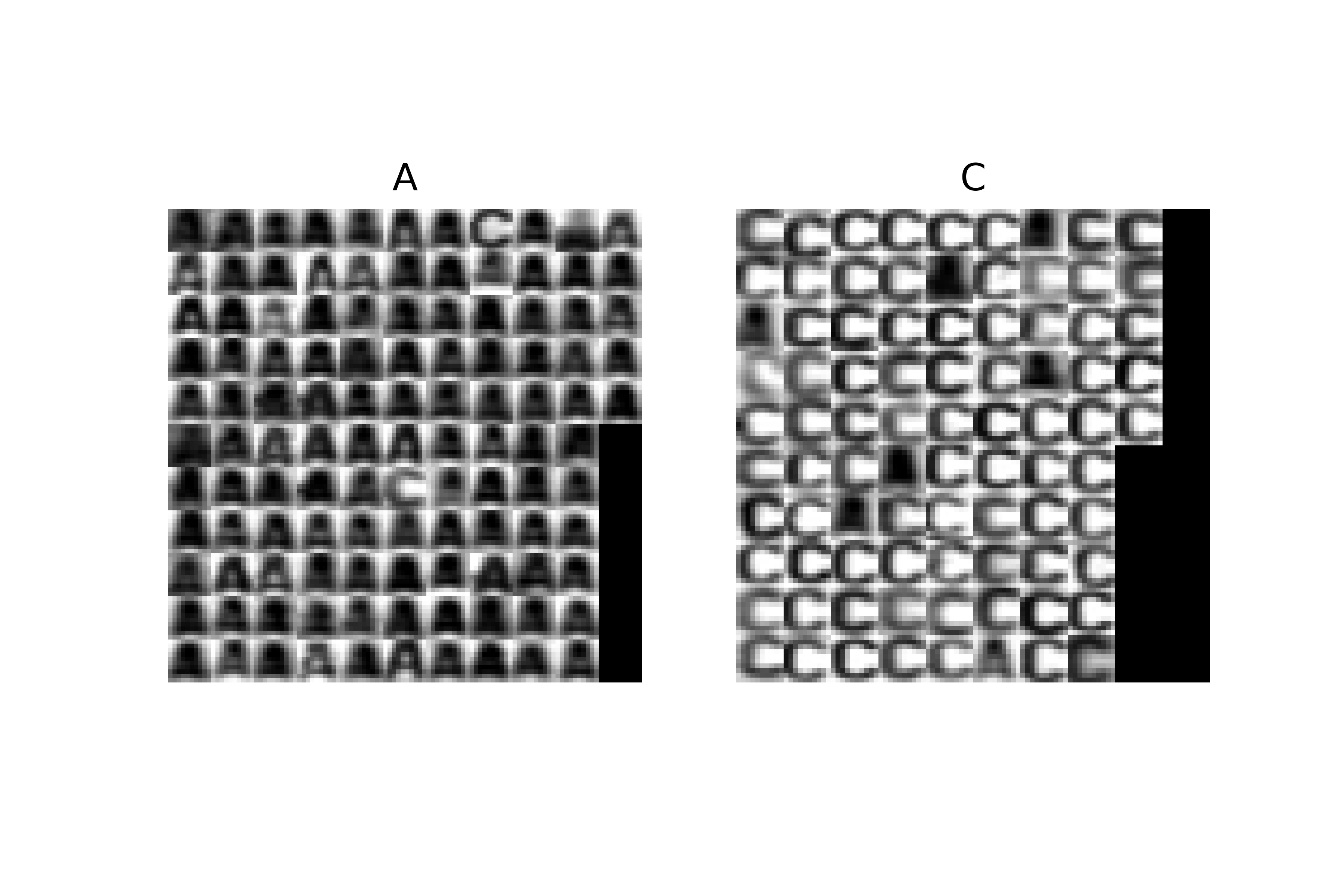## Assignment Questions

Fill the correct answers to your answers.txt file. For the following questions it is assumed that you run np.rand.seed(42) always before the splitting of the training set using crossval function. The dataset split will be unambiguously defined.

1. Imagine that some bad guy deleted all training data points except for the support vectors, but you still want to train SVM on this data. How will the absence of the majority of the training data points influence the resulting separating hyperplane compared to the one trained on full data?
• a) The absence of data points will have negative impact on the optimality.
• b) One cannot simply train SVM when some data points are missing.
• c) It will be exactly the same.
• d) The absence of data points will have positive impact on the optimality.
2. How can we enforce the soft-margin SVM to behave like a hard margin one (i.e. not allowing errors on training set)?
• a) Set value of $C$ to $0$.
• b) Set value of $C$ to $N$ (where $N$ is the size of the training set).
• c) It is not possible.
• d) Set value of $C$ to $\infty$.
3. What are the indices of the support vectors from task part 1 (point 4) for the case when training data are flawlessly classified into positive and negative class?
4. What is the optimal value of $C$ from the crossvalidation (Part 2, 2.IV)?
5. What is the classification error of the trained SVM on test data (Part 2, 4)?

Use MNIST data to create a multiclass classifier of images of numerals. The file contains 30 000 examples of normalized images of digits stored in matrix $X$ and corresponding labels (the label is the digit itself) $y \in \{0, 1, \dots, 9\}$. Use actual pixel values as features, in this case your feature space will be 784 dimensional.
Display the resulting classification (i.e. the montage of all images classified as $0, 1, \dots, 9$).